# 12.00 Bayesian Inference

Finally, let’s introduce the concept of Bayesian inference. Bayesian inference is a specific way to learn from data that is heavily used in statistics for data analysis. Bayesian inference is used less often in the field of machine learning, but it offers an elegant framework to understand what “learning” actually is. It is generally useful to know about Bayesian inference.

### Coin Flip Experiment

Before defining more formally what Bayesian inference is, let’s play a coin flipping game. Imagine that we have a bag of 100000 coins. When flipped, these coins randomly land on their heads or tails side. Each coin has a different probability ph of landing on its head that we are aware of (e.g. it is written on the coin). Let’s create such a fictional bag of coins:

We sampled the heads probabilities uniformly between 0 and 1 here. We can visualize these probabilities in a histogram:

The game that we are going to play is as follows. We put all these coins on a table and sort them according to their probabilities to visualize things better; coins with a small ph are on the left side of the table while those with a high ph are on the right side. Then, a friend secretly chooses one coin. The goal is to identify this particular coin, and its probability, by flipping all of the coins several times and asking our friend the outcomes for their chosen coin.

We first need to define a function to flip a coin given its heads probability:

Let’s start the game and flip all of the coins:

The table looks like this:

As expected, coins on the left tend to land on their tails side while the ones on the right tend to land on their heads side. Our friend informs us that their coin landed on its heads side. Therefore, we remove all the coins that landed on their tails side:

We now have about 50000 coins left. Let’s visualize their probabilities using a histogram (which corresponds to the density of blue points that we see on the table):

This histogram seems to follow a linear trend, which makes sense since one would expect around 10% of the coins that have ph0.1 to land on their heads, 20% of the coins that have ph0.2, and so on. At this stage, we don’t know yet which coin has been selected by our friend, but we know more about its ph value. Indeed, since the secret coin is one of these remaining coins, it makes sense to think that it has more of a chance of being biased toward heads than tails. We can go even further and say that the probability distribution for the ph value of the secret coin is given by this histogram. Of course, the secret coin has a defined ph value, so this probability distribution is relative to our knowledge. This is a sort of relative probability called Bayesian probability or simply belief.

Let’s now flip the remaining coins again:

Our friend informs us that their secret coin landed on tails this time. We therefore remove all coins that landed on heads and visualize the probability histogram for the remaining coins:

The histogram, which is equivalent to our belief about ph, is now symmetric. Let’s try to determine its shape mathematically. After the first selection, we determined that the shape was linear because only a fraction ph of each bin remained. In this step, we selected coins that landed on their tails, which means only a fraction 1-ph (the probability of tails) of each bin should remain. Since the initial bin counts were around 5000, the current bin value should be around 5000ph(1-ph):

This works pretty well. Let’s play a final round just to confirm. If the result of the secret coin is heads, one should expect to obtain a histogram that follows 5000ph2(1-ph):

This works well again. We could continue this game until we find the secret coin, but that is not the interesting part of this game. The game we played is actually equivalent to a more useful game: determining the value ph of a coin (no bag here) given its history of flipping results. Indeed, imagine that the secret coin is flipped in another room. Then, in effect, we are experimentally computing our belief about its ph value using our bag as a tool. Also, if the bag is infinite, we don’t need it anymore. We can just multiply our current belief by ph or 1-ph depending on the result of the coin to be identified. By doing so, we are effectively computing our belief in the ph value of the coin assuming that our original belief in ph is a uniform distribution between 0 and 1. This procedure is an example of Bayesian inference because we inferred (i.e. deduced) our belief about ph from our prior belief and the data.

### Bayesian Inference

In a general sense, Bayesian inference is a learning technique that uses probabilities to define and reason about our beliefs. In particular, this method gives us a way to properly update our beliefs when new observations are made. Let’s look at this more precisely in the context of machine learning. As an illustration, we will again use the problem of estimating the heads probability ph of a coin given its history of flipping results.

The usual goal of machine learning is to find a model that correctly represents or predicts the data (and specifically unseen data). Often, we have some knowledge about what this model could be even before seeing the data. The idea of Bayesian inference is to express this prior knowledge with a probability distribution over possible models called a prior distribution or prior belief. We write this prior distribution in the following way:

In our coin problem, the model is defined by a unique parameter ph, and our prior belief about this parameter could, for example, be a uniform distribution between 0 and 1:

Let’s now assume that we flip our coin once and that it lands on its heads side. We can update our belief to incorporate this new information (called observation or evidence in Bayesian terms). The updated belief is a called the posterior distribution, which we notate as: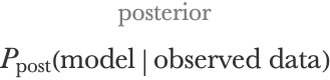The Bayesian inference procedure gives us a way to obtain (i.e. infer) this new belief, and it is simply done by multiplying the prior distribution by the likelihood function, notated as: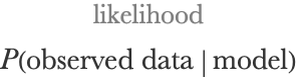The likelihood is the probability for a model to obtain this particular data, as defined in earlier chapters. After this multiplication, we still have to normalize the posterior distribution so that it sums to 1, but that is not the important part of this update. The overall belief update is given by: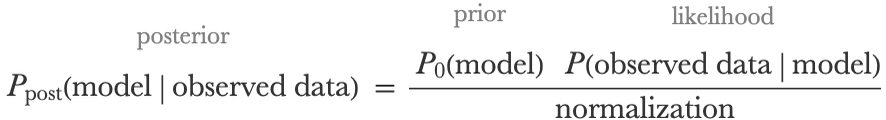This formula has many names, such as Bayes’s theorem, Bayes’s law, or Bayes’s rule, and it is the principal component of Bayesian inference. Note that this formula comes directly from probability theory: if A and B are two random variables, then P(A,B)=P(A|B)P(B) and similarly P(A,B)=P(B|A)P(A) (chain rule of probability), which means that:

And this is exactly the formula used to update our belief (P(B) is the normalization term). Note that this update can only be applied if the model is probabilistic (i.e. returns a predictive distribution instead of a pure prediction); otherwise, we could not compute a likelihood. An interesting aspect about using Bayesian inference to learn a model is that there is no cost function; the learning is purely done by reasoning about our belief and how new evidence modifies this belief.

Let’s apply this formula to our coin problem. If we first observe a heads, Bayes’s theorem gives: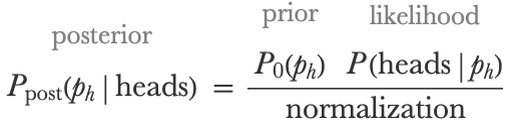Our prior is uniform in the interval [0,1], which means that P0(ph)=1 for all ph. Also, the likelihood for a heads result is simply P(heads|ph)=ph. Our unnormalized belief is then:

Which we can normalize:

Let’s visualize it:

If the next flip result is tails, we would have to multiply the belief by the probability for any model to land on tails, which is 1-ph, and we can continue this procedure as long as we want. If our coin landed 26 times on heads and 21 times on tails, our belief would be proportional to ph26(1-ph)21. Here is a program computing this belief for an arbitrary number of heads and tails:

This happens to be the density function of a beta distribution:

Let’s plot these beliefs for various flipping results:

We can see that our belief is changing as additional flips are performed. The uncertainty is reduced, and we are slowly narrowing down toward the true value of ph (and it is looking more and more like a normal distribution, in agreement with the central limit theorem). This acquisition of knowledge through observing data is a form of learning, and it can be used to think about other learning procedures as well.

Now that we have a probability distribution for ph, we can answer any question about the coin given our belief. For example, we could compute the probability of the coin being biased toward the heads side after the 21 tails and 26 heads recorded:

Or we could compute the probability, according to our belief, that it lands five times in a row on heads:

That is the basics of what Bayesian inference is. In the next section, we will look at how we can apply Bayesian inference to a predictive model.

### Bayesian Learning for Predictive Modeling

Let’s now see how we can apply Bayesian inference to something a bit more interesting to us, such as learning predictive models. Such Bayesian predictive models have the advantage of better modeling uncertainty than their non-Bayesian counterparts, which makes them useful for sensitive applications such as medicine.

We have already seen one example of Bayesian inference for predictive models in Chapter 10, Classic Supervised Learning Methods. Indeed, the Gaussian process method consists of conditioning a Gaussian process on the training data. Here is an illustration of this conditioning procedure (see the Gaussian Process section in Chapter 10 for more details):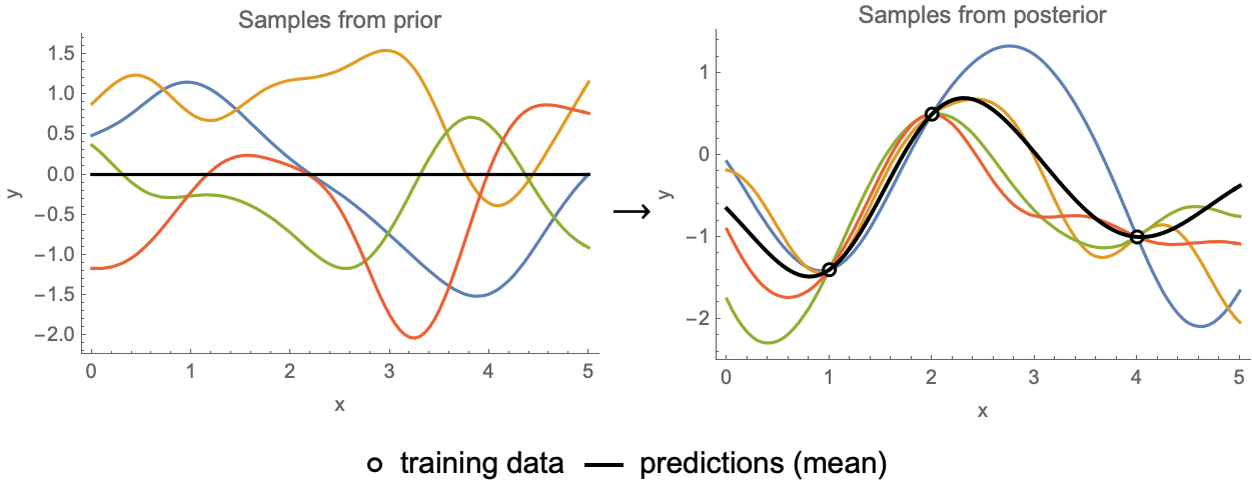On the left, we can see samples from a prior distribution over models, and on the right, we can see samples conditioned on the training data, which means samples from the posterior distribution. Such conditioning on the data is what Bayesian inference is. This is an example of nonparametric Bayesian inference because the model is nonparametric. Let’s now show the more classic case of Bayesian inference on a parametric model.

We again use the Car Stopping Distances dataset introduced in Chapter 4:

The goal is to predict the stopping distance as function of the speed. We first need to define a model suited for Bayesian learning. Previously, we used a class of models that directly returns predictions:

Here a and b are parameters and x is the speed. This model cannot be learned in a Bayesian way because it is not probabilistic; it needs to return a distribution instead of a unique value. To achieve this, we can wrap a normal distribution around the prediction. The model is now a conditional distribution Pa,b,σ(y|x) (y is the distance) that can be mathematically defined by: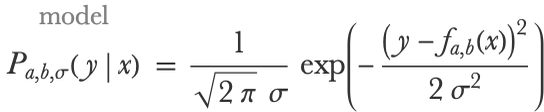Here σ is an additional learnable parameter corresponding to the standard deviation of the normal distribution. In a sense, σ is the amount of noise in the data (i.e. the fluctuations that are not predicted). This model can be defined by the following program:

Okay, so we want to train this model using a Bayesian procedure, which means that we want to deduce our posterior belief about the parameters given our prior belief and the data. We already defined a parametric class of models Pa,b,σ(y|x), which we will use to compute the likelihood term, so we now need to define our prior belief over these parameters.

In principle, the prior distribution should represent our belief about the model before we have looked at the data. That is, we should answer a question such as, “Amongst all hypothetical car stopping distance datasets that would have been recorded in 1930, what is, according to us, the probability distribution of the parameters a, b, and σ?” This is not an easy question to answer, and there are long-standing debates amongst statisticians about this topic. Fortunately, such a prior doesn’t need to be “perfect”; it just needs to be sensible. For simplicity, let’s first assume that the parameters are independent in the prior: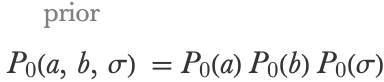We now only have to choose one prior for each parameter. To do so, let’s cheat a little bit and standardize the variables in the dataset:

Each variable is now centered (zero mean) and has a unit standard deviation. Such preprocessing should not really be done in a pure Bayesian treatment because the mean and variance are determined in a non-Bayesian way, but it is not a big problem in practice and will simplify the prior definition and the learning process. Since the data is standardized, we can assume that a is roughly between -1 and 1 and thus define its prior by a normal distribution with a unit standard deviation:

b should be closer to 0, so let’s assume a normal distribution with a standard deviation of 0.5:

For σ, which can be seen as the amount of noise, it is a bit more complicated. We know that it has to be positive and that it should be smaller than 1 because we standardized the data. We can also guess that amongst these hypothetical datasets, some will have large noise (e.g. 0.1<σ<1), but many others will have small noise (σ~0.01, σ~0.001, etc.), so we should allocate a decent probability fraction to small values. The following gamma distribution seems well suited:

This probability density has an exponential cutoff around 1, but more importantly, it is behaving like 1/σ0.5 near 0, which allows for fairly small values:

We can finally define our prior distribution over all parameters:

We have now defined everything we need to: a model and a prior. The next part is just computation. We are going to update our prior belief using the data in order to deduce what our posterior belief is. This deducing step is done by applying Bayes’s theorem, which in this case is: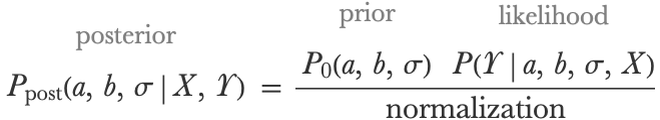Here X represents the training inputs and Y represents the training outputs. In some cases, it is possible to obtain a simple closed-form solution for this posterior distribution (like in the coin flip example where the posterior is a beta distribution), but in general, it is not possible. The good news is that there is a way out; we can generally obtain samples from the posterior distribution, which means obtaining a set of models as if they were generated by the posterior distribution. This set of models can be seen as an approximation of the posterior distribution, and they can be used to make predictions, which we will see.

The most classic method to sample from a posterior distribution is the Markov chain Monte Carlo method (often called MCMC). The idea of this sampling method is to randomly modify an initial model (a set of parameters here) several times in a specific way so that the model eventually becomes a valid sample from the desired distribution. This procedure can be seen as a random walk in the space of parameters, which is called a Markov chain. The trick is that these modifications are random but biased toward models with higher posterior (quite like an optimization procedure). In our case, we are using the classic Metropolis algorithm where we “propose” small random modifications (also, reversible and uniform) but only accept them with the probability:

This means that sometimes a modification is rejected and a copy of the current model is used instead. Note that a modification that increases the posterior distribution is always accepted here. Here is an illustration of what such a chain could look like for sampling a normal distribution: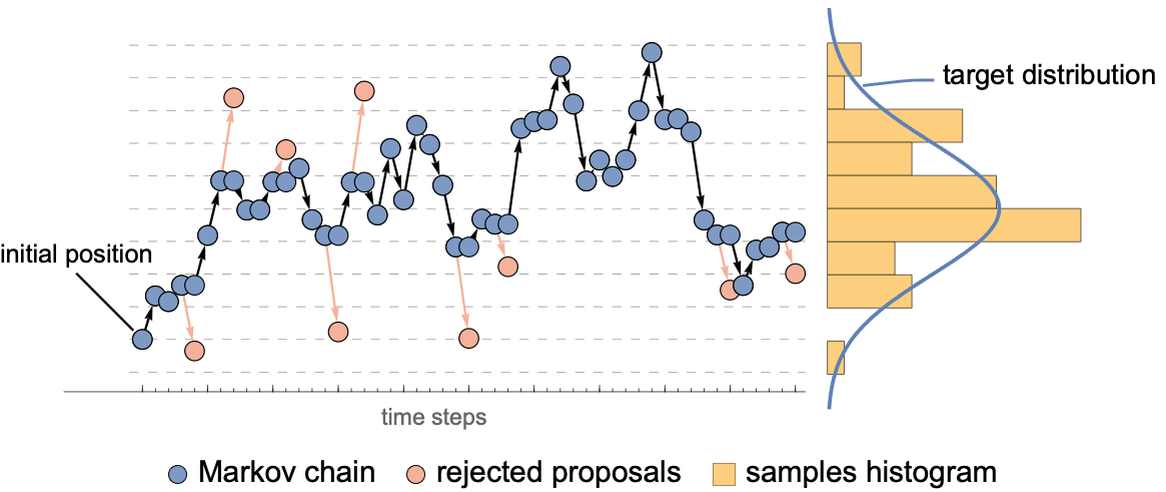This procedure can be shown to converge toward samples from the desired distribution. One important thing to note is that since we only need to compute distribution ratios, we don’t need to know the normalization constant of the posterior (which can be difficult to obtain).

Okay, let’s implement this for the linear regression problem. We first need a function to compute the likelihood:

Note that we compute the log of the likelihood to avoid numeric precision problems. Then, we need something to compute the (log) PDF of the prior:

Finally, let’s define how the posterior is computed from the prior and the likelihood. Since we don’t care about the normalization constant here, and since we work in log-space, we just need to sum the log-prior and the log-likelihood:

We now need to implement a program to perform an MCMC simulation:

Let’s now perform 300 consecutive modifications starting from the initial parameters {a,b,σ}={-1,1,1}:

We obtained a chain of 301 sets of parameters (in a sense 301 models), and here are the first five iterations:

The chain drifts away from its initial position. Let’s visualize the parameters {a,b} of this chain displayed on top of the posterior for σ=1 (for reference):

We can see two regimes. During the first ~50 steps, the chain drifts toward regions of high posterior, like an optimization process would. Then the chain enters a stationary regime where it wanders around the maximum of the posterior. We are only interested in this stationary regime, so let’s discard the transient steps:

We now have 250 models left, which represent models sampled from the posterior distribution. Let’s compare prior and posterior distributions for the parameter a by doing a histogram of its values in the chain:

Our belief in parameter a is now around 0.8. As expected, the uncertainty of our belief diminished because we learned from the data. Consecutive models in the chain tend to be similar to each other, so there is no point keeping all of them. Let’s reduce the size of the chain further by selecting one model every 10 steps of the chain:

We now have our final 25 models, which we will use to make predictions. Let’s first visualize the predictions of all these models and compare them with models sampled from the prior:

As expected, samples from the posterior fit the data while samples from the prior do not necessarily fit the data. We can interpret the Bayesian inference procedure as a selecting of the models from the prior that fit the data. Note that if we had less data, the variability of our posterior samples would be greater (and lower if we had more data).

Okay, we now need to make predictions, which means that we need to combine all these models into a unique predictive model. A simple solution could be, for example, to take the mean value of each parameter a, b, and σ, which is known as the posterior mean:

We could also take the most likely parameters according to the posterior, something known as the maximum a posteriori (MAP) estimate:

Both of these so-called “point estimate” solutions give a unique parametric model to work with, which is handy but is not as good as using the full posterior distribution over parameters. The proper way to create a predictive Bayesian model is to average the predictive distributions of every model under the posterior distribution, which can be written as follows: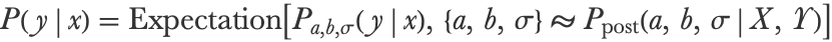This gives the predictive distribution P(y|x) of the combined model for a given input x. We can compute an approximation of this distribution by using our posterior samples. The resulting predictive distribution is a mixture of distributions made by single models:

Let’s visualize this distribution for a standardized speed of x=1.3:

In this case, it looks like a normal distribution, but it does not have to be, even if the original model is returning a normal distribution. Let’s now visualize the predictions made by the median of this distribution along with a 66% confidence interval:

We can see that the result is pretty close to a vanilla linear regression. In this case, the benefits of a Bayesian approach are not apparent. If we reduce the number of examples, it becomes clearer. Here is what we obtain when we only have three training examples: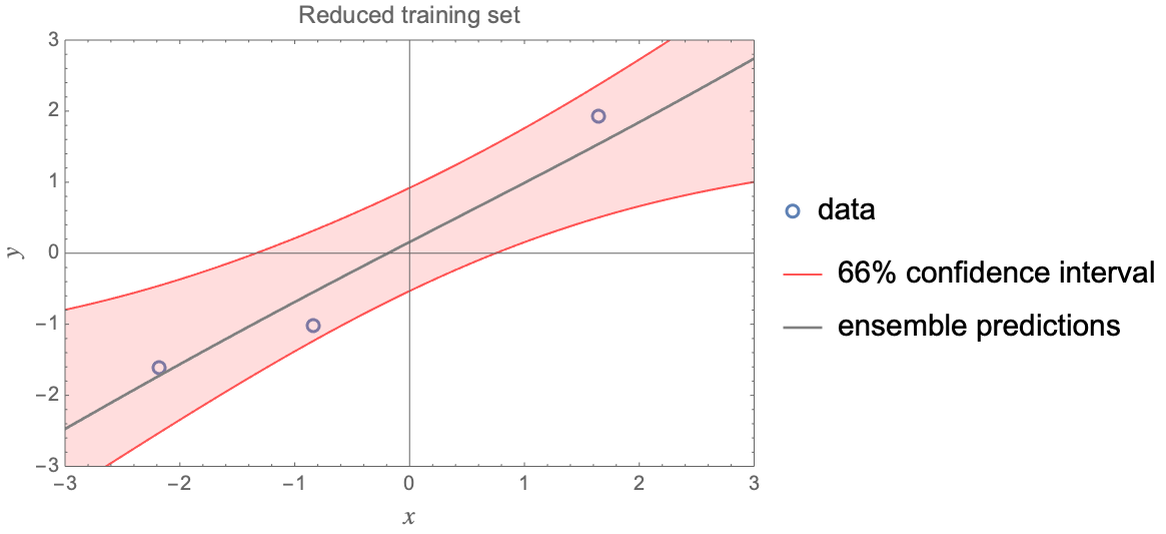We can see that the resulting noise is not uniform, for example, the uncertainty increases as we go out of the domain. Providing good “lack-of-examples” uncertainties is one of the advantages of Bayesian learning. The other advantage of Bayesian approaches is that they allow for the precise definition of our prior knowledge about a problem. This is particularly useful if the data is scarce and if we have expert knowledge about the problem. For example, we could know which variables interact together and their causal relations. Adding prior knowledge to a problem improves the performance of the learned model, at least if the knowledge is correct. The downside of Bayesian inference is that expressing our prior knowledge as a distribution is often difficult, and it might not even be very useful if we have a lot of data.

This is how we can use Bayesian inference to train and use predictive models. Here we applied Bayesian inference to the simple model of linear regression, but it can also be applied to more complex parametric models such as neural networks. Learning Bayesian neural networks is not that complicated. Like in this example, we would use a Markov chain instead of an optimization procedure and obtain an ensemble of networks from which we can combine predictions. Obtaining a unique sample from a Markov chain is not much slower than training a usual neural network. Bayesian neural networks typically obtain better results and provide better predictive distributions than regular networks. One downside is that we need to handle several networks, and it is not clear which prior to use. Also, as it happens, simply training several networks independently in a non-Bayesian way (e.g. by just using different random seeds) often leads to equivalent results. Overall, Bayesian neural network are, as of the early 2020s, more of a domain of research than a heavily used method.

### Probabilistic Programming

In the previous section, we implemented a Bayesian linear regression from scratch. In practice, we would use a tool for this task, and a powerful kind of tool is called probabilistic programming. The idea of probabilistic programming is to use a regular programming language to define distributions, which can then be conditioned to perform a Bayesian inference. Let’s look at this in more detail.

There are two main ways to define a distribution: either by defining its probability density function (PDF) or by defining a sampling procedure. Simple numeric distributions are usually defined by their PDF. For example, we can construct a normal distribution in the following way:

We can then use this distribution to obtain random samples:

Of course, we can also use this distribution to compute probability densities:

We could define higher-dimensional distributions in a similar manner.

A related way to define distributions is by using an energy function instead of the PDF. This energy function E(u1,u2,...) implicitly defines an unnormalized PDF through the relation PDF(u1,u2,...)exp(-E(u1,u2,...)). In principle, we can always compute the normalization constant if we want to (in practice, it can be difficult), which means that this energy function is enough to fully define a distribution. Models that define, in one way or another, a probability distribution through their energy function are called energy-based models.

Defining a distribution through its PDF or energy function has its applications but is not the preferred way in the context of Bayesian inference. Instead, distributions are generally defined by their sampling process. The idea of probabilistic programming is to express this sampling process using a regular programming language. To explain this, let’s go back to the coin flip problem and let’s solve it in a probabilistic programming style.

The first thing to do is write a probabilistic program that simulates the full coin flipping experiment according to our prior belief. Let’s say that our prior over ph is uniform and that we throw the coins 10 times. Such a program could be:

This program defines a distribution and we can use it to generate one (or more) realization of our experiment according to our belief:

Here "Outcomes" and "p" are the two random variables of our distribution. Note that most of the outcomes are tails here because the program sampled a low value for "p" (ph). Now, to perform a Bayesian inference, we simply need to condition this distribution on the actual outcomes:

There are currently (early 2021) no probabilistic programming capabilities in the Wolfram Language, but such a operation would look like this:

Then, we could sample from this conditioned distribution several times and plot a histogram of the result, which would look like this: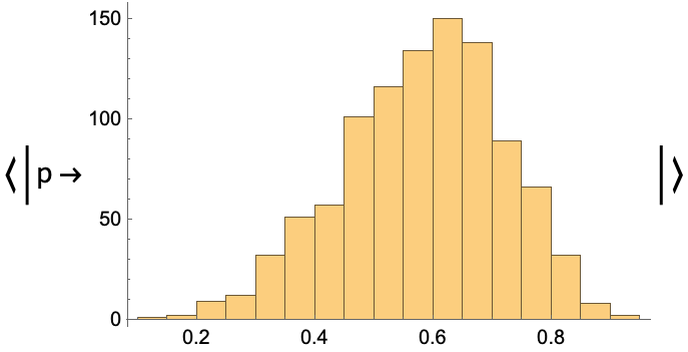This would give an estimation of our posterior belief in the value of ph, which is what we are interested in. Under the hood, this sampling would use an MCMC procedure. The program responsible for generating the samples is called an inference engine and might involve using automatic differentiation of the probabilistic program like a neural network would do.

That is pretty much what the probabilistic programming procedure is. Variations might include tweaking the parameters of the inference engine. Note that, conceptually, this procedure is even simpler than the traditional view of Bayesian inference because we don’t need to define parameters, priors, likelihood, or a normalization term here. We only need to define a distribution that generates both the data and the parameters of interest and then condition this distribution on the actual data. We could use this procedure to perform a Bayesian linear regression or just about any other Bayesian inference task.

Probabilistic programs are descendent of probabilistic graphical models, which are distributions defined by graphs. The probabilistic program that we wrote could also be implemented through a directed graph called a Bayesian network. The advantage of programs over graphical models is that they offer more flexibility (we can use conditional statements, loops, etc.) and are generally simpler to implement. On the other hand, a graph offers a nice visualization of the model.

This probabilistic programming/graphical approach is heavily used in statistics, mainly to analyze experimental data in a Bayesian way. It is also used to perform causal inference, such as obtaining the distribution of a variable after intervening on the value of another variable. Such causal inference is a central problem in statistics and might be a driver for a wider adoption of probability programming.

Probabilistic programming is also used in machine learning, although not as much as in statistics. A classic machine learning example of a probabilistic graph/program is the latent Dirichlet allocation (LDA) model, which is the standard way to model topics in text documents. Before the rise of deep learning in the 2010s, it was also thought that such approaches would be the solution for tackling perception tasks like computer vision. The idea here is to use a probabilistic model to simulate the world and infer its latent variables (object types, positions, etc.), which is the vision as inverse graphics approach. As of the early 2020s, neural networks are still the main way to solve such tasks. Overall, probabilistic programming is used for some machine learning applications, but it is not dominant (yet?). In any case, Bayesian inference is always useful for helping us think about how machine learning works.

Takeaways
 ■ Bayesian inference is a way to learn from data by combining explicit prior knowledge with the data. ■ Prior knowledge is defined by a prior distribution over possible models. ■ Learning means deducing the posterior distribution of models given the data. ■ Bayesian models are good at expressing their uncertainty, which is necessary for sensitive applications. ■ Bayesian learning allows for the specification of prior knowledge precisely, therefore requiring less data to learn. ■ Expressing our prior knowledge as a distribution can be difficult. ■ Probabilistic programming and probabilistic graphical models are the usual tools for performing Bayesian inference. ■ Bayesian inference is a classic method for statistical data analysis. ■ Bayesian inference is used in machine learning for some applications, such as topic modeling.
Vocabulary
Exercises
Coin flip
12.1Continue the coin flip game with a few extra flips.
12.2Compare the resulting histogram with the theoretical distribution.
12.3Plot the theoretical distribution for 582 heads and 254 tails.
12.4Change the prior and see how it affects the result.
Bayesian linear regression
12.5Change the prior distributions of the parameters and see how it affects the predictions and uncertainties.
12.6Try to obtain posterior samples by sampling from the prior and (correctly) selecting some of the samples. Think of the advantages and disadvantages of this method over a Markov chain approach.
12.7Write a probabilistic program to generate data from a Bayesian linear regression model.